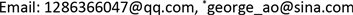﻿ Atkinson类型的具有分布势函数的Sturm-Liouville问题的逆谱问题 Inverse Sturm-Liouville Problems with Distribution Potentials of Atkinson Type

Vol. 07  No. 12 ( 2018 ), Article ID: 28103 , 9 pages
10.12677/AAM.2018.712183

Inverse Sturm-Liouville Problems with Distribution Potentials of Atkinson Type

Liang Zhang, Jijun Ao*

College of Sciences, Inner Mongolia University of Technology, Hohhot Inner MongoliaReceived: Nov. 21st, 2018; accepted: Dec. 13th, 2018; published: Dec. 20th, 2018ABSTRACT

In this paper, the inverse Sturm-Liouville problems with distribution potentials of Atkinson type are studied. We use the conclusions of the inverse eigenvalue problems of Jacobi matrix and cyclic Jacobi matrix to obtain the corresponding inverse Sturm-Liouville problems with distribution potentials which have a finite spectrum.

Keywords:Inverse Spectral Problems, Sturm-Liouville Problems, Distribution Potentials, Inverse Eigenvalue Problems

Atkinson类型的具有分布势函数的Sturm-Liouville问题的逆谱问题1. 引言

2015年，闫军在其博士论文中详细介绍了具有分布势函数的S-L问题的有限谱理论  ，给出了具有分布势函数的S-L问题的矩阵表示。2016年，唐松林在其硕士论文中也对具有分布势函数的S-L问题的多种带有转移条件的情况进行研究  。近年来，具有分布势函数的S-L问题引起了一大批数学工作者的关注与讨论，相关成果可参见     。

2. 预备知识

$VX=\lambda WX,$ (1)

$-{\left(p\left[{y}^{\prime }+sy\right]\right)}^{\prime }+sp\left[{y}^{\prime }+sy\right]+qy=\lambda wy,\text{\hspace{0.17em}}\text{\hspace{0.17em}}I=\left(a,b\right),\text{\hspace{0.17em}}\text{\hspace{0.17em}}-\infty (2)

$r=\frac{1}{p},q,w,s\in L\left(I,ℝ\right),$ (3)

$-{\left({y}^{\left[1\right]}\right)}^{\prime }+s{y}^{\left[1\right]}+qy=\lambda wy,\text{\hspace{0.17em}}\text{\hspace{0.17em}}I=\left(a,b\right),\text{\hspace{0.17em}}\text{\hspace{0.17em}}-\infty

$AY\left(a\right)+BY\left(b\right)=0,\text{\hspace{0.17em}}\text{\hspace{0.17em}}Y={\left[y,{y}^{\left[1\right]}\right]}^{\text{T}},\text{\hspace{0.17em}}\text{\hspace{0.17em}}A,B\in {M}_{2}\left(ℝ\right),$ (4)

$rank\left(A,B\right)=2,\text{\hspace{0.17em}}\text{\hspace{0.17em}}AE{A}^{*}=BE{B}^{*},\text{\hspace{0.17em}}\text{\hspace{0.17em}}E=\left[\begin{array}{cc}0& -1\\ 1& 0\end{array}\right],$

$\begin{array}{l}\mathrm{cos}\alpha y\left(a\right)-\mathrm{sin}\alpha {y}^{\left[1\right]}\left(a\right)=0,\text{\hspace{0.17em}}\text{\hspace{0.17em}}0\le \alpha <\text{π},\\ \mathrm{cos}\beta y\left(b\right)-\mathrm{sin}\beta {y}^{\left[1\right]}\left(b\right)=0,\text{\hspace{0.17em}}\text{\hspace{0.17em}}0<\beta \le \text{π};\end{array}$ (5)

$Y\left(b\right)=kY\left(a\right),\text{\hspace{0.17em}}\text{\hspace{0.17em}}K=\left({k}_{ij}\right)\in S{L}_{2}\left(ℝ\right),$ (6)

$a={a}_{0}<{a}_{1}<{a}_{2}<\cdots <{a}_{n}=b,$ (7)

$\begin{array}{l}在\left[{a}_{2i},{a}_{2i+1}\right]上,\text{\hspace{0.17em}}\text{\hspace{0.17em}}r=s=0,\text{\hspace{0.17em}}\text{\hspace{0.17em}}i=0,1,\cdots ,m,\\ {\int }_{{a}_{2i+1}}^{{a}_{2i+2}}{\text{e}}^{2{\int }_{{a}_{2i+1}}^{t}s\left(u\right)\text{d}u}r\left(t\right)\text{d}t>0,\text{\hspace{0.17em}}\text{\hspace{0.17em}}{\int }_{{a}_{2i+1}}^{{a}_{2i+2}}s\left(t\right)\text{d}t\ne 0,\text{\hspace{0.17em}}\text{\hspace{0.17em}}i=0,1,\cdots ,m-1;\end{array}$ (8)

$在\left[{a}_{2i+1},{a}_{2i+2}\right]上,\text{\hspace{0.17em}}\text{\hspace{0.17em}}q=0,\text{\hspace{0.17em}}\text{\hspace{0.17em}}i=0,1,\cdots ,m-1;$ (9)

$\begin{array}{l}在\left[{a}_{2i+1},{a}_{2i+2}\right]上,\text{\hspace{0.17em}}\text{\hspace{0.17em}}\text{\hspace{0.17em}}w=0,\text{\hspace{0.17em}}\text{\hspace{0.17em}}i=1,2,\cdots ,m-1,\\ {\int }_{{a}_{2i}}^{{a}_{2i+1}}w\left(t\right)\text{d}t>0,\text{\hspace{0.17em}}\text{\hspace{0.17em}}i=0,1,\cdots ,m.\end{array}$ (10)

3. 具有分布势函数的Sturm-Liouville问题的矩阵表示

$\begin{array}{l}{r}_{2i+1}={\int }_{{a}_{2i+1}}^{{a}_{2i+2}}{\text{e}}^{2{\int }_{{a}_{2i+1}}^{t}s\left(u\right)\text{d}u}r\left(t\right)\text{d}t>0,\text{\hspace{0.17em}}\text{\hspace{0.17em}}{s}_{2i+1}={\int }_{{a}_{2i+1}}^{{a}_{2i+2}}s\left(t\right)\text{d}t\ne 0,\text{\hspace{0.17em}}\text{\hspace{0.17em}}i=0,1,\cdots ,m-1,\\ {q}_{2i}={\int }_{{a}_{2i}}^{{a}_{2i+1}}q\left(t\right)\text{d}t,\text{\hspace{0.17em}}\text{\hspace{0.17em}}{w}_{2i}={\int }_{{a}_{2i}}^{{a}_{2i+1}}w\left(t\right)\text{d}t,\text{\hspace{0.17em}}\text{\hspace{0.17em}}i=0,1,\cdots ,m.\end{array}$ (11)

$\stackrel{¯}{p}\left(t\right)=\left\{\begin{array}{l}\frac{{a}_{2i+2}-{a}_{2i+1}}{{r}_{2i+1}{s}_{2i+1}},\text{\hspace{0.17em}}\text{\hspace{0.17em}}t\in \left[{a}_{2i+1},{a}_{2i+2}\right],\text{\hspace{0.17em}}\text{\hspace{0.17em}}i=0,1,\cdots ,m-1;\\ \infty ,\text{\hspace{0.17em}}\text{\hspace{0.17em}}t\in \left[{a}_{2i},{a}_{2i+1}\right],\text{\hspace{0.17em}}\text{\hspace{0.17em}}i=0,1,\cdots ,m,\end{array}\text{ }\left({s}_{2i+1}\ne 0\right),$

$\stackrel{¯}{p}\left(t\right)=\left\{\begin{array}{l}\frac{{a}_{2i+2}-{a}_{2i+1}}{{r}_{2i+1}},\text{\hspace{0.17em}}\text{\hspace{0.17em}}t\in \left[{a}_{2i+1},{a}_{2i+2}\right],\text{\hspace{0.17em}}\text{\hspace{0.17em}}i=0,1,\cdots ,m-1;\\ \infty ,\text{\hspace{0.17em}}\text{\hspace{0.17em}}t\in \left[{a}_{2i},{a}_{2i+1}\right],\text{\hspace{0.17em}}\text{\hspace{0.17em}}i=0,1,\cdots ,m,\end{array}\text{ }\left({s}_{2i+1}=0\right),$

$\stackrel{¯}{s}\left(t\right)=\left\{\begin{array}{l}\frac{{s}_{2i+1}}{{a}_{2i+2}-{a}_{2i+1}},\text{\hspace{0.17em}}\text{\hspace{0.17em}}t\in \left[{a}_{2i+1},{a}_{2i+2}\right],\text{\hspace{0.17em}}\text{\hspace{0.17em}}i=0,1,\cdots ,m-1;\\ 0,\text{\hspace{0.17em}}\text{\hspace{0.17em}}t\in \left[{a}_{2i},{a}_{2i+1}\right],\text{\hspace{0.17em}}\text{\hspace{0.17em}}i=0,1,\cdots ,m,\end{array}$

$\stackrel{¯}{q}\left(t\right)=\left\{\begin{array}{l}\frac{{q}_{2i}}{{a}_{2i+1}-{a}_{2i}},\text{\hspace{0.17em}}\text{\hspace{0.17em}}t\in \left[{a}_{2i},{a}_{2i+1}\right],\text{\hspace{0.17em}}\text{\hspace{0.17em}}i=0,1,\cdots ,m;\\ 0,\text{\hspace{0.17em}}\text{\hspace{0.17em}}t\in \left[{a}_{2i+1},{a}_{2i+2}\right],\text{\hspace{0.17em}}\text{\hspace{0.17em}}i=0,1,\cdots ,m-1,\end{array}$

$\stackrel{¯}{w}\left(t\right)=\left\{\begin{array}{l}\frac{{w}_{2i}}{{a}_{2i+1}-{a}_{2i}},\text{\hspace{0.17em}}\text{\hspace{0.17em}}t\in \left[{a}_{2i},{a}_{2i+1}\right],\text{\hspace{0.17em}}\text{\hspace{0.17em}}i=0,1,\cdots ,m;\\ 0,\text{\hspace{0.17em}}\text{\hspace{0.17em}}t\in \left[{a}_{2i+1},{a}_{2i+2}\right],\text{\hspace{0.17em}}\text{\hspace{0.17em}}i=0,1,\cdots ,m-1.\end{array}$

$-{\left(\stackrel{¯}{p}\left[{y}^{\prime }+\stackrel{¯}{s}y\right]\right)}^{\prime }+\stackrel{¯}{s}\stackrel{¯}{p}\left[{y}^{\prime }+\stackrel{¯}{s}y\right]+\stackrel{¯}{q}y=\lambda \stackrel{¯}{w}y,\text{\hspace{0.17em}}\text{\hspace{0.17em}}I=\left(a,b\right),$ (12)

${P}_{\alpha \beta }=\left[\begin{array}{ccccc}\frac{1}{{r}_{1}}+\mathrm{cot}\alpha & -\frac{{\text{e}}^{{s}_{1}}}{{r}_{1}}& & & \\ -\frac{{\text{e}}^{{s}_{1}}}{{r}_{1}}& \frac{{\text{e}}^{2{s}_{1}}}{{r}_{1}}+\frac{1}{{r}_{3}}& -\frac{{\text{e}}^{{s}_{3}}}{{r}_{3}}& & \\ & \cdots & \cdots & \cdots & \\ & & -\frac{{\text{e}}^{{s}_{2m-3}}}{{r}_{2m-3}}& \frac{{\text{e}}^{2{s}_{2m-3}}}{{r}_{2m-3}}+\frac{1}{{r}_{2m-1}}& -\frac{{\text{e}}^{{s}_{2m-1}}}{{r}_{2m-1}}\\ & & & -\frac{{\text{e}}^{{s}_{2m-1}}}{{r}_{2m-1}}& \frac{{\text{e}}^{2{s}_{2m-1}}}{{r}_{2m-1}}-\mathrm{cot}\beta \end{array}\right],$ (13)

$\begin{array}{l}{Q}_{\alpha \beta }=diag\left({q}_{0},{q}_{2},\cdots ,{q}_{2m-2},{q}_{2m}\right),\\ {W}_{\alpha \beta }=diag\left({w}_{0},{w}_{2},\cdots ,{w}_{2m-2},{w}_{2m}\right).\end{array}$ (14)

$\begin{array}{l}{\left({P}_{\alpha \beta }+{Q}_{\alpha \beta }\right)}_{1}={P}_{0\beta }+{Q}_{0\beta },\text{\hspace{0.17em}}\text{\hspace{0.17em}}{\left({P}_{\alpha \beta }+{Q}_{\alpha \beta }\right)}^{1}={P}_{\alpha \pi }+{Q}_{\alpha \pi },\\ {\left({P}_{\alpha \pi }+{Q}_{\alpha \pi }\right)}_{1}={P}_{0\pi }+{Q}_{0\pi },\text{\hspace{0.17em}}\text{\hspace{0.17em}}{\left({P}_{0\beta }+{Q}_{0\beta }\right)}^{1}={P}_{0\pi }+{Q}_{0\pi }.\end{array}$

${P}_{I}=\left[\begin{array}{ccccc}\frac{1}{{r}_{1}}-\frac{{k}_{11}}{{k}_{12}}& -\frac{{\text{e}}^{{s}_{1}}}{{r}_{1}}& & & \frac{1}{{k}_{12}}\\ -\frac{{\text{e}}^{{s}_{1}}}{{r}_{1}}& \frac{{\text{e}}^{2{s}_{1}}}{{r}_{1}}+\frac{1}{{r}_{3}}& -\frac{{\text{e}}^{{s}_{3}}}{{r}_{3}}& & \\ & \cdots & \cdots & \cdots & \\ & & -\frac{{\text{e}}^{{s}_{2m-3}}}{{r}_{2m-3}}& \frac{{\text{e}}^{2{s}_{2m-3}}}{{r}_{2m-3}}+\frac{1}{{r}_{2m-1}}& -\frac{{\text{e}}^{{s}_{2m-1}}}{{r}_{2m-1}}\\ \frac{1}{{k}_{12}}& & & -\frac{{\text{e}}^{{s}_{2m-1}}}{{r}_{2m-1}}& \frac{{\text{e}}^{2{s}_{2m-1}}}{{r}_{2m-1}}-\frac{{k}_{22}}{{k}_{12}}\end{array}\right],$ (15)

$\begin{array}{l}{Q}_{I}=diag\left({q}_{0},{q}_{2},\cdots ,{q}_{2m-2},{q}_{2m}\right),\\ {W}_{I}=diag\left({w}_{0},{w}_{2},\cdots ,{w}_{2m-2},{w}_{2m}\right).\end{array}$ (16)

${P}_{\theta }=\left[\begin{array}{ccccc}-{k}_{11}{k}_{21}+\frac{1}{{r}_{1}}+{k}_{11}^{2}\frac{{\text{e}}^{{s}_{2m-1}}}{{r}_{2m-1}}& -\frac{{\text{e}}^{{s}_{1}}}{{r}_{1}}& & & -{k}_{11}\frac{{\text{e}}^{{s}_{2m-1}}}{{r}_{2m-1}}\\ -\frac{{\text{e}}^{{s}_{1}}}{{r}_{1}}& \frac{{\text{e}}^{2{s}_{1}}}{{r}_{1}}+\frac{1}{{r}_{3}}& -\frac{{\text{e}}^{{s}_{3}}}{{r}_{3}}& & \\ & \cdots & \cdots & \cdots & \\ & & -\frac{{\text{e}}^{{s}_{2m-5}}}{{r}_{2m-5}}& \frac{{\text{e}}^{2{s}_{2m-5}}}{{r}_{2m-5}}+\frac{1}{{r}_{2m-3}}& -\frac{{\text{e}}^{{s}_{2m-3}}}{{r}_{2m-3}}\\ -{k}_{11}\frac{{\text{e}}^{{s}_{2m-1}}}{{r}_{2m-1}}& & & -\frac{{\text{e}}^{{s}_{2m-3}}}{{r}_{2m-3}}& \frac{{\text{e}}^{2{s}_{2m-3}}}{{r}_{2m-3}}+\frac{1}{{r}_{2m-1}}\end{array}\right],$ (17)

$\begin{array}{l}{Q}_{\theta }=diag\left({q}_{0}+{k}_{11}^{2}{q}_{2m},{q}_{2},\cdots ,{q}_{2m-2}\right),\\ {W}_{\theta }=diag\left({w}_{0}+{k}_{11}^{2}{w}_{2m},{w}_{2},\cdots ,{w}_{2m-2}\right).\end{array}$ (18)

4. 矩阵的逆特征值问题

$J=\left[\begin{array}{ccccc}{c}_{1}& {d}_{1}& & & \\ {d}_{1}& {c}_{2}& {d}_{2}& & \\ & \cdots & \cdots & \cdots & \\ & & {d}_{k-2}& {c}_{k-1}& {d}_{k-1}\\ & & & {d}_{k-1}& {c}_{k}\end{array}\right].$ (19)

${\lambda }_{1}<{\mu }_{1}<{\lambda }_{2}<{\mu }_{2}<\cdots <{\lambda }_{k-1}<{\mu }_{k-1}<{\lambda }_{k}.$ (20)

$W=diag\left({w}_{1},\cdots ,{w}_{k}\right)$ 是一个对角矩阵，其中 ${w}_{i}>0,i=1,\cdots ,k$ 。则存在唯一的负Jacobi矩阵 $M\in {\mathbb{M}}_{k}$ 使得： $\sigma \left(M,W\right)=\left\{{\lambda }_{i}:i=1,\cdots ,k\right\}$$\sigma \left({M}_{1},{W}_{1}\right)=\left\{{\mu }_{i}:i=1,\cdots ,k-1\right\}$

${J}_{c}=\left[\begin{array}{ccccc}{c}_{1}& {d}_{1}& & & {d}_{k}\\ {d}_{1}& {c}_{2}& {d}_{2}& & \\ & \cdots & \cdots & \cdots & \\ & & {d}_{k-2}& {c}_{k-1}& {d}_{k-1}\\ {d}_{k}& & & {d}_{k-1}& {c}_{k}\end{array}\right].$ (21)

i) ${\lambda }_{1}\le {\mu }_{1}\le {\lambda }_{2}\le {\mu }_{2}\le \cdots \le {\lambda }_{k-1}\le {\mu }_{k-1}\le {\lambda }_{k}$

ii) ${\mu }_{i}\ne {\mu }_{j}$$i\ne j$

iii) $\exists d>0$ ，对于 $j=1,\cdots ,k-1$

$\underset{i=1}{\overset{k}{\prod }}|{\mu }_{j}-{\lambda }_{i}|\ge 2d\left[1+{\left(-1\right)}^{k+1-j}\right].$ (22)

$W=diag\left({w}_{1},\cdots ,{w}_{k}\right)$ 是一个对角矩阵，其中 ${w}_{i}>0,\text{\hspace{0.17em}}i=1,\cdots ,k$ 。则存在唯一的负循环Jacobi矩阵 $N\in {\mathbb{M}}_{k}$ ，使得 ${\prod }_{i=1}^{k}{d}_{i}=d$ ，并且： $\sigma \left(N,W\right)=\left\{{\lambda }_{i}:i=1,\cdots ,k\right\}$$\sigma \left({N}_{1},{W}_{1}\right)=\left\{{\mu }_{i}:i=1,\cdots ,k-1\right\}$

5. 主要结论及其证明

a) 存在函数 $r,q\in L\left(I,ℝ\right)$ 满足(8)和(9)使得S-L问题(2)，(5)及其等价类的谱为

$\sigma \left(\alpha ,\beta \right)=\left\{{\lambda }_{i}:i=1,\cdots ,k\right\},\text{\hspace{0.17em}}\text{\hspace{0.17em}}\sigma \left(0,\beta \right)=\left\{{\mu }_{i}:i=1,\cdots ,k-1\right\}.$

b) 存在函数 $r,q\in L\left(I,ℝ\right)$ 满足(8)和(9)使得S-L问题(2)，(5)及其等价类的谱为

$\sigma \left(\alpha ,\beta \right)=\left\{{\lambda }_{i}:i=1,\cdots ,k\right\},\text{\hspace{0.17em}}\text{\hspace{0.17em}}\sigma \left(\alpha ,\text{π}\right)=\left\{{\mu }_{i}:i=1,\cdots ,k-1\right\}.$

${s}_{2i+1}={\int }_{{a}_{2i+1}}^{{a}_{2i+2}}s\left(t\right)\text{d}t,\text{\hspace{0.17em}}\text{\hspace{0.17em}}i=0,1,\cdots ,m-1,\text{\hspace{0.17em}}\text{\hspace{0.17em}}\text{\hspace{0.17em}}{w}_{2i}={\int }_{{a}_{2i}}^{{a}_{2i+1}}w\left(t\right)\text{d}t,\text{\hspace{0.17em}}\text{\hspace{0.17em}}i=0,1,\cdots ,m,$

${W}_{\alpha \beta }=diag\left({w}_{0},{w}_{2},\cdots ,{w}_{2m}\right).$

$\sigma \left(M,W\right)=\left\{{\lambda }_{i}:i=1,\cdots ,m+1\right\},\text{\hspace{0.17em}}\text{\hspace{0.17em}}\sigma \left({M}_{1},{W}_{1}\right)=\left\{{\mu }_{i}:i=1,\cdots ,m\right\}.$

$\begin{array}{l}{r}_{2i-1}=-\frac{{\text{e}}^{{s}_{2i-1}}}{{d}_{i}},\text{\hspace{0.17em}}i=1,\cdots ,m;\text{\hspace{0.17em}}\text{\hspace{0.17em}}\text{\hspace{0.17em}}{q}_{0}={c}_{1}-\frac{1}{{r}_{1}}-\mathrm{cot}\alpha ,\\ {q}_{2i}={c}_{i+1}-\frac{{\text{e}}^{2{s}_{2i-1}}}{{r}_{2i-1}}-\frac{1}{{r}_{2i-1}},\text{\hspace{0.17em}}i=1,\cdots ,m-1,\text{\hspace{0.17em}}\text{\hspace{0.17em}}\text{\hspace{0.17em}}{q}_{2m}={c}_{m+1}-\frac{{\text{e}}^{2{s}_{2m-1}}}{{r}_{2m-1}}+\mathrm{cot}\beta .\end{array}$

$\begin{array}{l}\sigma \left({P}_{\alpha \beta }+{Q}_{\alpha \beta },{W}_{\alpha \beta }\right)=\left\{{\lambda }_{i}:i=1,\cdots ,m+1\right\},\\ \sigma \left({P}_{0\beta }+{Q}_{0\beta },{W}_{0\beta }\right)=\left\{{\mu }_{i}:i=1,\cdots ,m\right\}.\end{array}$

$\sigma \left(\alpha ,\beta \right)=\left\{{\lambda }_{i}:i=1,\cdots ,m+1\right\},\text{\hspace{0.17em}}\text{\hspace{0.17em}}\sigma \left(0,\beta \right)=\left\{{\mu }_{i}:i=1,\cdots ,m\right\}.$

b) 证明方法与a)相同，只需利用推论4.1，引理4.1，引理3.2和注3.1即可，故省略证明细节。

$m=k-1$ 。则对于区间 $I=\left[a,b\right]$ $\left(-\infty 上的任何划分(7)，任何函数 $s\in L\left(I,ℝ\right)$ 满足(8)以及任何函数 $w\in L\left(I,ℝ\right)$ 满足(10)，我们有以下结论：

a) 对于 $\forall \beta \in \left(0,\text{π}\right)$$\exists K=\left({k}_{ij}\right)\in S{L}_{2}\left(ℝ\right)$ 满足 ${k}_{12}<0$$\mathrm{cot}\beta ={k}_{22}/{k}_{12}$ ，并且存在函数 $r,q\in L\left(I,ℝ\right)$ 满足(8)和(9)，使得S-L问题(2)，(6)及其等价类的谱为

$\sigma \left(K\right)=\left\{{\lambda }_{i}:i=1,\cdots ,k\right\},\text{\hspace{0.17em}}\text{\hspace{0.17em}}\sigma \left(0,\beta \right)=\left\{{\mu }_{i}:i=1,\cdots ,k-1\right\}.$

b) 对于 $\forall \alpha \in \left(0,\text{π}\right)$$\exists K=\left({k}_{ij}\right)\in S{L}_{2}\left(ℝ\right)$ 满足 ${k}_{12}<0$$\mathrm{cot}\alpha =-{k}_{11}/{k}_{12}$ ，并且存在函数 $r,q\in L\left(I,ℝ\right)$ 满足(8)和(9)，使得S-L问题(2)，(6)及其等价类的谱为

$\sigma \left(K\right)=\left\{{\lambda }_{i}:i=1,\cdots ,k\right\},\text{\hspace{0.17em}}\text{\hspace{0.17em}}\sigma \left(\alpha ,\pi \right)=\left\{{\mu }_{i}:i=1,\cdots ,k-1\right\}.$

${s}_{2i+1}={\int }_{{a}_{2i+1}}^{{a}_{2i+2}}s\left(t\right)\text{d}t,\text{\hspace{0.17em}}i=0,1,\cdots ,m-1,\text{\hspace{0.17em}}\text{\hspace{0.17em}}\text{\hspace{0.17em}}{w}_{2i}={\int }_{{a}_{2i}}^{{a}_{2i+1}}w\left(t\right)\text{d}t,\text{\hspace{0.17em}}i=0,1,\cdots ,m,$

${W}_{I}=diag\left({w}_{0},{w}_{2},\cdots ,{w}_{2m}\right).$

$\sigma \left(N,W\right)=\left\{{\lambda }_{i}:i=1,\cdots ,m+1\right\},\text{\hspace{0.17em}}\text{\hspace{0.17em}}\sigma \left({N}_{1},{W}_{1}\right)=\left\{{\mu }_{i}:i=1,\cdots ,m\right\}.$

$\begin{array}{l}{q}_{0}={c}_{1}-\frac{1}{{r}_{1}}+\frac{{k}_{11}}{{k}_{12}}，\text{\hspace{0.17em}}\\ {q}_{2i}={c}_{i+1}-\frac{{\text{e}}^{2{s}_{2i-1}}}{{r}_{2i-1}}-\frac{1}{{r}_{2i-1}},\text{\hspace{0.17em}}i=1,\cdots ,m-1,\\ {q}_{2m}={c}_{m+1}-\frac{{\text{e}}^{2{s}_{2m-1}}}{{r}_{2m-1}}+\frac{{k}_{22}}{{k}_{12}}.\end{array}$

$\begin{array}{l}\sigma \left({P}_{I}+{Q}_{I},{W}_{I}\right)=\left\{{\lambda }_{i}:i=1,\cdots ,m+1\right\},\\ \sigma \left({P}_{0\beta }+{Q}_{0\beta },{W}_{0\beta }\right)=\left\{{\mu }_{i}:i=1,\cdots ,m\right\}.\end{array}$

$\sigma \left(K\right)=\left\{{\lambda }_{i}:i=1,\cdots ,m+1\right\},\text{\hspace{0.17em}}\text{\hspace{0.17em}}\sigma \left(0,\beta \right)=\left\{{\mu }_{i}:i=1,\cdots ,m\right\}.$

b) 证明方法与a)相同，利用推论4.3，引理4.2，3.2，3.3和注3.1即可。证明细节略。

$\sigma \left(K\right)=\left\{{\lambda }_{i}:i=1,\cdots ,k\right\},\text{\hspace{0.17em}}\text{\hspace{0.17em}}\sigma \left(0,\text{π}\right)=\left\{{\mu }_{i}:i=1,\cdots ,k-1\right\}.$

Inverse Sturm-Liouville Problems with Distribution Potentials of Atkinson Type[J]. 应用数学进展, 2018, 07(12): 1565-1573. https://doi.org/10.12677/AAM.2018.712183

1. 1. Atkinson, F.V. (1964) Discrete and Continuous Boundary Problems. Academic Press, New York/London.

2. 2. Kong, Q., Wu, H. and Zettl, A. (2001) Sturm-Liouville Problems with Finite Spectrum. Journal of Mathematical Analysis and Applications, 263, 748-762. https://doi.org/10.1006/jmaa.2001.7661

3. 3. Volkmer, H. and Zettl, A. (2007) Inverse Spectral Theory for Sturm-Liouville Problems with Finte Spectrum. Proceedings of the American Mathematical Society, 135, 1129-1132. https://doi.org/10.1090/S0002-9939-06-08563-7

4. 4. Kong, Q., Volkmer, H. and Zettl, A. (2009) Matrix Representations of Sturm-Liouville Problems with Finite Spectrum. Results in Mathematics, 54, 103-116. https://doi.org/10.1007/s00025-009-0371-3

5. 5. Kong, Q. and Zettl, A. (2012) Inverse Sturm-Liouville Problems with Finite Spectrum. Journal of Mathematical Analysis and Applications, 386, 1-9. https://doi.org/10.1016/j.jmaa.2011.06.083

6. 6. Xu, S.F. (1998) An Introduction to Inverse Algebraic Eigenvalue Problems. Peking University Press, Beijing.

7. 7. 阎军. 具有分布势函数的Sturm-Liouville问题的谱性质[D]: [博士学位论文]. 天津: 天津大学, 2015.

8. 8. 唐松林. 具有分布势函数和转移条件的Sturm-Liouville问题的谱性质[D]: [博士学位论文]. 曲阜: 曲阜师范大学, 2016.

9. 9. Hryniv, R.O. and Mykytyuk, Ya.V. (2003) Inverse Spectral Problems for Sturm-Liouville Operators with Singular Potentials. Part III: Reconstruction by Three Spectra. Journal of Mathematical Analysis and Applications, 284, 626-646. https://doi.org/10.1016/S0022-247X(03)00370-6

10. 10. Savchuk, A.M. and Shkalikov, A.A. (2005) Inverse Prob-lem for Sturm-Liouville Operators with Distribution Potentials: Reconstruction from Two Spectra. Russian Journal of Mathematical Physics, 12, 507-514.

11. 11. Savchuk, A.M. and Shkalikov, A.A. (2003) Sturm-Liouville Operators with Distribution Potentials. Transactions of the Moscow Mathematical Society, 64, 143-192.

12. 12. Savchuk, A.M. and Shkalikov, A.A. (2006) On the Eigenvalues of the Sturm-Liouville Operator with Potentials from Sobolev Spaces. Mathematical Notes, 80, 814-832. https://doi.org/10.1007/s11006-006-0204-6